﻿ Some Curvature Properties on a Special Paracontact Kenmotsu Manifold with Respect to Semi-Symmetric Connection

### Some Curvature Properties on a Special Paracontact Kenmotsu Manifold with Respect to Semi-Symmetric ...

K. L. Sai Prasad, T. Satyanarayana

Turkish Journal of Analysis and Number Theory

## Some Curvature Properties on a Special Paracontact Kenmotsu Manifold with Respect to Semi-Symmetric Connection

K. L. Sai Prasad1,, T. Satyanarayana2

1Department of Mathematics, Gayatri Vidya Parishad College of Engineering for Women, Visakhapatnam, Andhra Pradesh, India

2Department of Mathematics, Pragathi Engineering College, Surampalem, Near Peddapuram, Andhra Pradesh, India

### Abstract

The object of the present paper is to study some properties of curvature tensor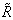of a semi-symmetric non-metric connection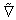in a type of special paracontact Kenmotsu (briefly SP-Kenmotsu) manifold. We have deduced the expressions for curvature tensorand the Ricci tensor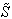of Mn with respect to semi-symmetric non-metric connection. It is proved that in an SP-Kenmotsu manifold if the curvature tensor of the semi-symmetric non-metric connection vanishes then the manifold is projectively flat.

• K. L. Sai Prasad, T. Satyanarayana. Some Curvature Properties on a Special Paracontact Kenmotsu Manifold with Respect to Semi-Symmetric Connection. Turkish Journal of Analysis and Number Theory. Vol. 3, No. 4, 2015, pp 94-96. http://pubs.sciepub.com/tjant/3/4/1
• Prasad, K. L. Sai, and T. Satyanarayana. "Some Curvature Properties on a Special Paracontact Kenmotsu Manifold with Respect to Semi-Symmetric Connection." Turkish Journal of Analysis and Number Theory 3.4 (2015): 94-96.
• Prasad, K. L. S. , & Satyanarayana, T. (2015). Some Curvature Properties on a Special Paracontact Kenmotsu Manifold with Respect to Semi-Symmetric Connection. Turkish Journal of Analysis and Number Theory, 3(4), 94-96.
• Prasad, K. L. Sai, and T. Satyanarayana. "Some Curvature Properties on a Special Paracontact Kenmotsu Manifold with Respect to Semi-Symmetric Connection." Turkish Journal of Analysis and Number Theory 3, no. 4 (2015): 94-96.

 Import into BibTeX Import into EndNote Import into RefMan Import into RefWorks

### 1. Introduction

Friedmann and Schouten [1, 2] introduced the idea of semi-symmetric linear connection on a differentiable manifold. Hayden  introduced semi-symmetric metric connection on a Riemannian manifold and it was further developed by Yano . Semi-symmetric connections play an important role in the study of Riemannian manifolds. There are various physical problems involving the semi-symmetric metric connection. For example, if a man is moving on the surface of the earth always facing one definite point, say Jaruselam or Mekka or the North pole, then this displacement is semi-symmetric and metric . In 1975, Prvanovi´c  introduced the concept of semi-symmetric non-metric connection with the name pseudo-metric, which was further studied by Andonie [6, 7]. The study of semi-symmetric non-metric connection is much older than the nomenclature ’non-metric’ was introduced. In 1992, Agashe and Chafle  introduced a semi-symmetric connection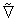satisfying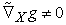on a Riemannian manifold, and called such a connection as semi-symmetric non-metric connection. Later, the curvature properties of the connection in an SP-Sasakian manifold were studied by Bhagwat Prasad , and many others.

On the other hand, in 1976, Sato  defined the notions of an almost paracontact Riemannian manifold. After that, T. Adati and K. Matsumoto  defined and studied para-Sasakian and SP-Sasakian manifolds which are regarded as a special kind of an almost contact Riemannian manifolds. Before Sato, in 1972, Kenmotsu  defined a class of almost contact Riemannian manifolds satisfying some special conditions. In 1995, Sinha and Sai Prasad  have defined a class of almost paracontact metric manifolds namely para Kenmotsu (briefly P-Kenmotsu) and special para Kenmotsu (briefly SP-Kenmotsu) manifolds.

In 1970, Pokhariyal and Mishra  have introduced new tensor fields, called W and E-tensor fields in a Riemannian manifold and studied their properties. In the present paper, we consider the W-curvature tensor of a semi-symmetric non-metric connection and obtained a relation connecting the curvature tensors of Mn with respect to semi-symmetric non-metric connection and the Riemannian connection. It is proved that in an SP-Kenmotsu manifold if the curvature tensor of the semi-symmetric non-metric connection vanishes then the manifold is protectively flat.

Let Mn be an n-dimensional differentiable manifold equipped with structure tensors (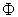, ξ, η) whereis a tensor of type (1, 1), ξ is a vector field, η is a 1-form such that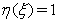(1.1)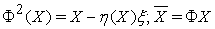(1.2)

Then Mn is called an almost paracontact manifold.

Let g be the Riemannian metric in an n-dimensional almost paracontact manifold Mn such that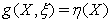(1.3)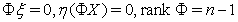(1.4)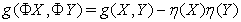(1.5)

for all vector fields X and Y on Mn. Then the manifold Mn  is said to admit an almost paracontact Riemannian structure (, ξ, η, g) and the manifold is called an almost paracontact Riemannian manifold.

A manifold Mn with Riemannian metric ‘g’ admitting a tensor fieldof type (1, 1), a vector field ξ and 1-form η satisfying equations (1.1), (1.3) along with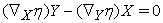(1.6)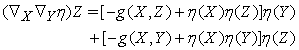(1.7)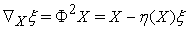(1.8)

is called a para Kenmotsu manifold or briefly P-Kenmotsu manifold , where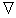is the covariant differentiation with respect to g.

It is known that  on a P-Kenmotsu manifold the following relations hold: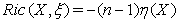(1.9)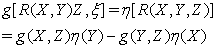(1.10)

where R is the Riemannian curvature.

Let (Mn, g) be an n-dimensional Riemannian manifold admitting a tensor fieldof type (1, 1), a vector field ξ and 1-form η satisfying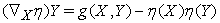(1.11)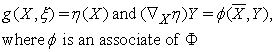(1.12)

is called a special para Kenmotsu manifold or briefly SP-Kenmotsu manifold .

A linear connectionin a Riemannian manifold Mn is said to be semi-symmetric connection if its torsion tensor T satisfies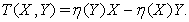(1.13)

A semi-symmetric non-metric connectionin an almost paracontact metric manifold with torsion tensor (1.13) is given by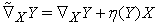(1.14)

whereis a Riemannian connection with respect to metric g .

Apart from conformal curvature tensor, the projective curvature tensor is an other important tensor from the differential geometric point of view. The Weyl-projective curvature tensor W of type (1, 3) of a Riemannian manifold Mn with respect to the Riemannian connection is defined by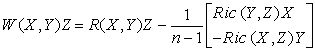(1.15)

for X, Y, Z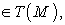where R is the curvature tensor and Ric is the Ricci tensor. If there exists a one-to-one correspondence between each coordinate neighbourhood of a Riemannian manifold Mn and a domain in Eucledian space such that any geodesic of the Riemannian manifold corresponds to a straight line in the Eucledian space, then Mn is said to be locally projectively flat. For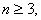Mn is locally projectively flat if and only if the projective curvature tensor W vanishes. For n = 2, the projective curvature tensor identically vanishes.

### 2. Curvature Tensor

The manifold Mn is considered to be an SP-Kenmotsu manifold. Let us denote the curvature tensor of the semi-symmetric non-metric connectionby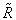and the curvatre tensor ofby R. By straight forward calculation, we get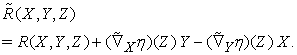(2.1)

As a consequence of equations (1.11) and (1.14), equation (2.1) reduces to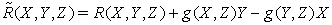(2.2)

which is the relation between the curvature tensors of Mn with respect to the semi-symmetric non-metric connectionand the Riemannian connection.

It is well known that a Riemannian manifold is of constant curvature if and only if it is projectively flat or conformally flat  and in general, the necessary and sufficient condition for a manifold with a symmetric linear connection to be projectively flat is that the projective curvature tensor with respect to it vanishes identically on a manifold .

As an example, if Mn is a Riemannian manifold with vanishing curvature tensor with respect to semi-symmetric non-metric connection, then Mn is projectively flat . Analogus to this, we prove the following for an SP-Kenmotsu manifold which is Riemannian.

Theorem 2.1: If in an SP-Kenmotsu manifold Mn the curvature tensor of a semi-symmetric non-metric connectionvanishes, then the manifold is projectively flat.

Proof: Since= 0, then equation (2.2) gives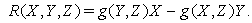(2.3)

On contracting the above equation, we get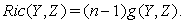(2.4)

Then, by (2.3) and (2.4), we get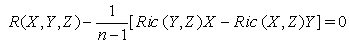(2.5)

or W = 0 from (1.15), proves that the manifold is projectively flat.

Theorem 2.2: If in an SP-Kenmotsu manifold the Ric tensor of a semi-symmetric non-metric connectionvanishes, then the curvature tensor ofis equal to the projective curvature tensor of the manifold Mn.

Proof: From equation (2.2), we have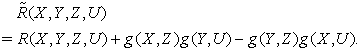(2.6)

On contracting the above equation, we get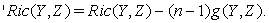(2.7)

Since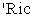= 0, we have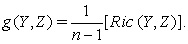(2.8)

From equations (2.2) and (2.8), we have= W.

Theorem 2.3: In an SP-Kenmotsu manifold the projective curvature tensor of a semi-symmetric non-metric connectionis equal to the projective curvature tensor of the manifold.

Proof: From equations (2.2) and (2.7), we get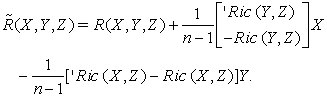(2.9)

The terms of the equation (2.9) can be rearranged as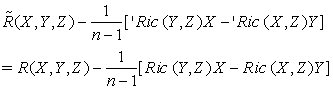(2.10)

which is ‘W = W, where ‘W is the Weyl projective curvature tensor with respect to the semi-symmetric non-metric connection.

Theorem 2.4: In an SP-Kenmotsu manifold with semi-symmetric non-metric connectionwe have

a)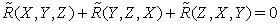b)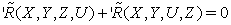Proof: Using the Bianchi’s first identity with respect to the Riemannian connection equation (2.2) gives (a). From equation (2.6) we get (b).

### Acknowledgement

The authors acknowledge Prof. Kalpana, Banaras Hindu University, Dr. B. Satyanarayana of Nagarjuna University and Dr. A. Kameswara Rao, G.V.P. College of Engineering for Women for their valuable suggestions in preparation of the manuscript. They are also thankful to the referee for his valuable comments in the improvement of this paper.

### Competing Interest

The authors declare that there is no conflict of interests regarding the publication of this paper.

### References

  Friedmann and Schouten, J. A., Uber die Geometrie der halbsymmetrischen Ubertragungen, Math Zeitschrift, 21, 211-223, 1924.In article View Article  Schouten, J. A., Ricci-calculus, Springer-Verlag. Berlin, 1954.In article View Article  PubMed  Hayden, H. A., Subspaces of a space with torsion, Proc. London Math. Soc., 34, 27-50, 1932.In article View Article  Yano, K., On semi-symmetric metric connection, Revue Roumanine de Mathematiques Pures et Appliques, 15, 1579-1581, 1970.In article  Prvanovi´c, M., On pseudo metric semi-symmetric connections, Pub. De L’Institut Math., N.S., 18(32), 157-164, 1975.In article  Andonie, P.O.C., On semi-symmetric non-metric connection on a Riemannian manifold. Ann. Fac. Sci. De Kinshasa, Zaire sect. Math. Phys., 2, 1976.In article  Andonie, P.O.C., Smaranda, D., Certains connections semi-symmetriques, Tensor (N.S.), 31, 8-12, 1977.In article  Agashe, N. S. and Chafle, M. R., A semi-symmetric non-metric connection on a Riemannian manifold, Ind. J. of Pure and Appl. Math., 23(6), 399-409, 1992.In article  Prasad, On a semi-symmetric non-metric connection in an sp-Sasakian manifold, Istambul Univ.Fen Fak. Mat. Der., 53, 77-80, 1994.In article  Sato, I., On a structure similar to the almost contact structure, Tensor (N.S.), 30, 219-224, 1976.In article  Adati, T. and Matsumoto, K., On conformally recurrent and conformally symmetric P-Sasakian manifolds, TRU Math., 13, 25-32, 1977.In article  Kenmotsu, K., A class of almost contact Riemannian manifolds, Tohoku Math. Journal, 24, 93-103, 1972.In article View Article  Sinha, B. B. and Sai Prasad, K. L., A class of almost para contact metric Manifold, Bulletin of the Calcutta Mathematical Society, 87, 307-312, 1995.In article  Pokhariyal, G.P., Mishra, R.S., The curvature tensors and their relativistic significance, Yokohoma Math.J., 18, 105-108, 1970.In article  Yano, K. and Bochner, S., Curvature and Betti numbers, Annals of Math Studies 32, Princeton University Press, 1953.In article  Sinha, B. B., An introduction to modern Differential Geometry, Kalyani Publishers, New Delhi, 1982.In article